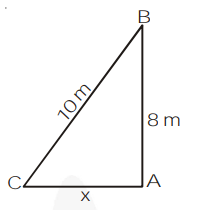# A ladder 10 m long reaches a window 8 m above the ground.

Question.

A ladder 10 m long reaches a window 8 m above the ground. Find the distance of the foot of the ladder from base of the wall

Solution:

Let AC = x metres be the distance of the foot of the ladder from the base of the wall.AB = 8 m (Height of window)

BC = 10 m (length of ladder)

Now, $\quad x^{2}+(8)^{2}=(10)^{2}$

$\Rightarrow x^{2}=100-64=36 \Rightarrow x=6$, i.e., $A C=6 m$